##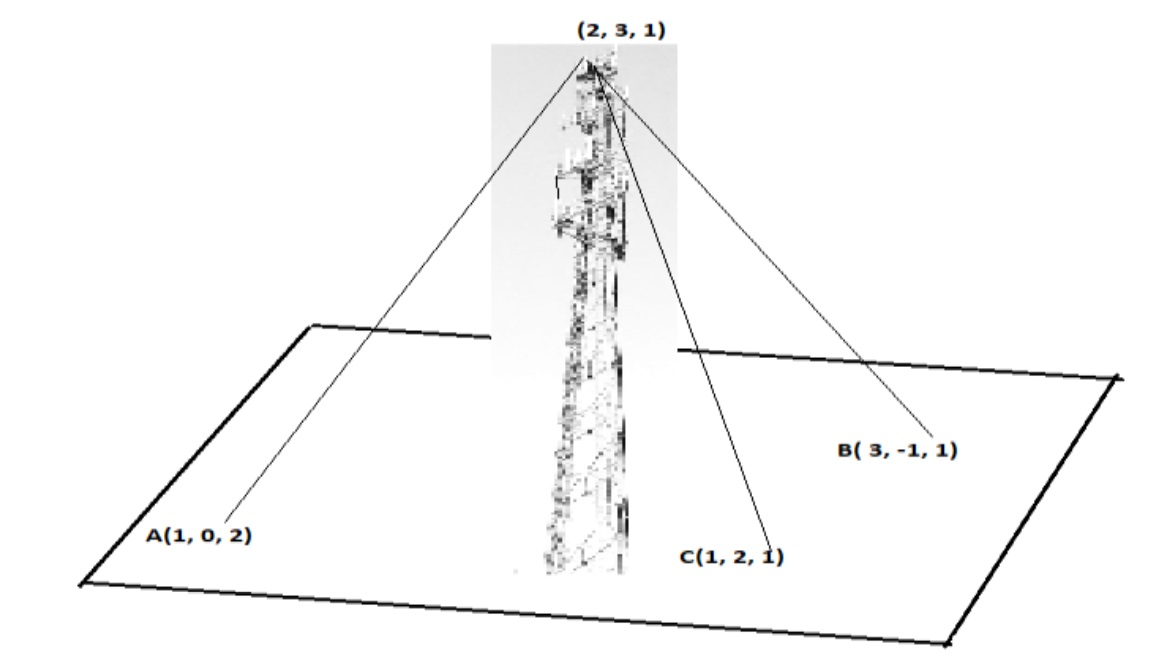(adsbygoogle = window.adsbygoogle || []).push({}); Based on the above answer the following: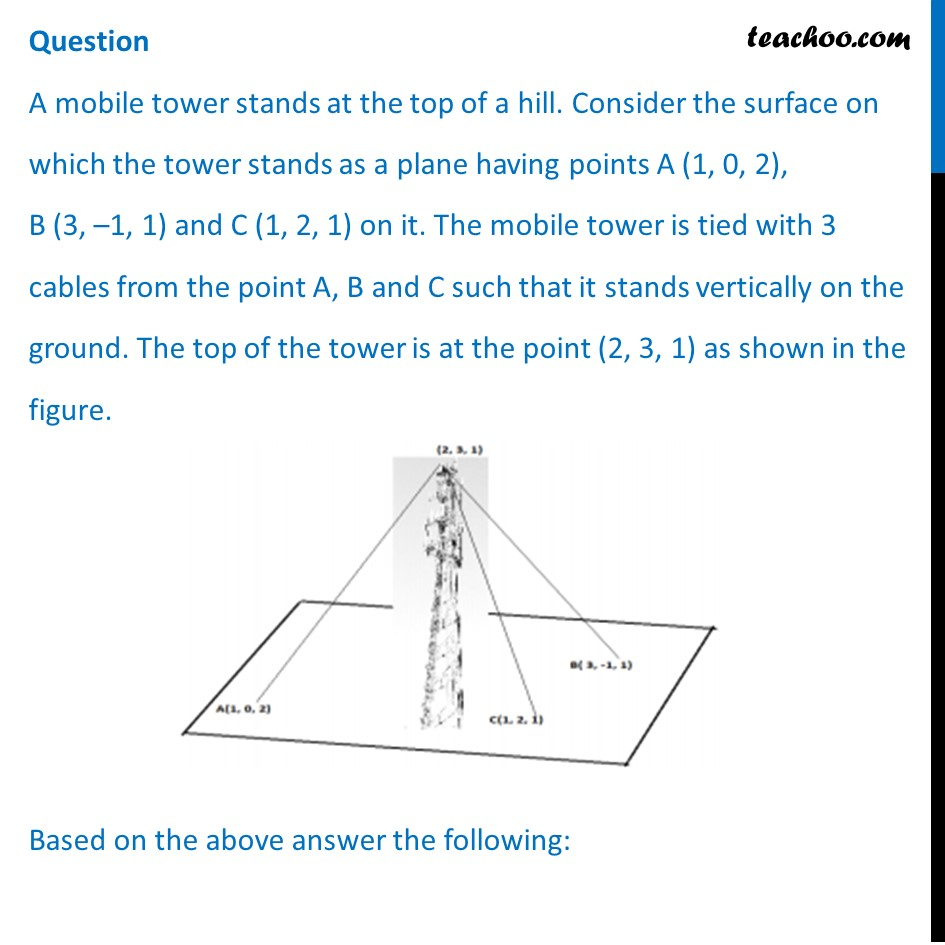## (d) –3x + 2y + 4z = –11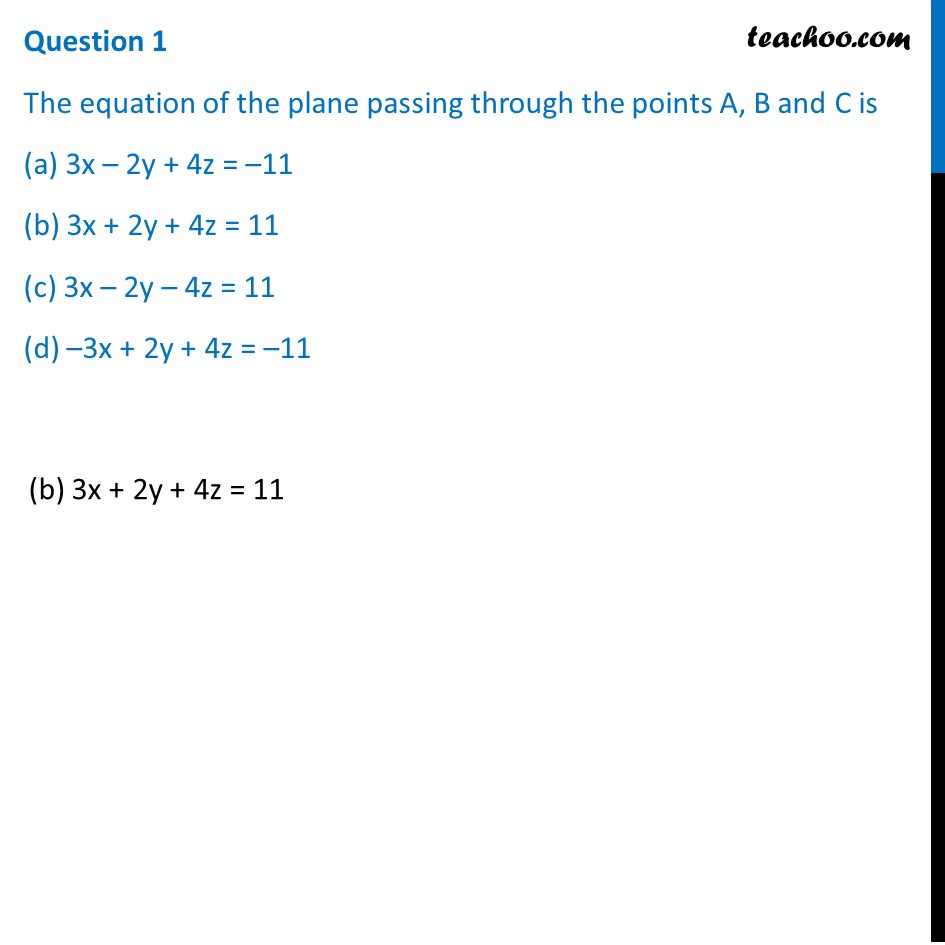## (d) 8/√29 units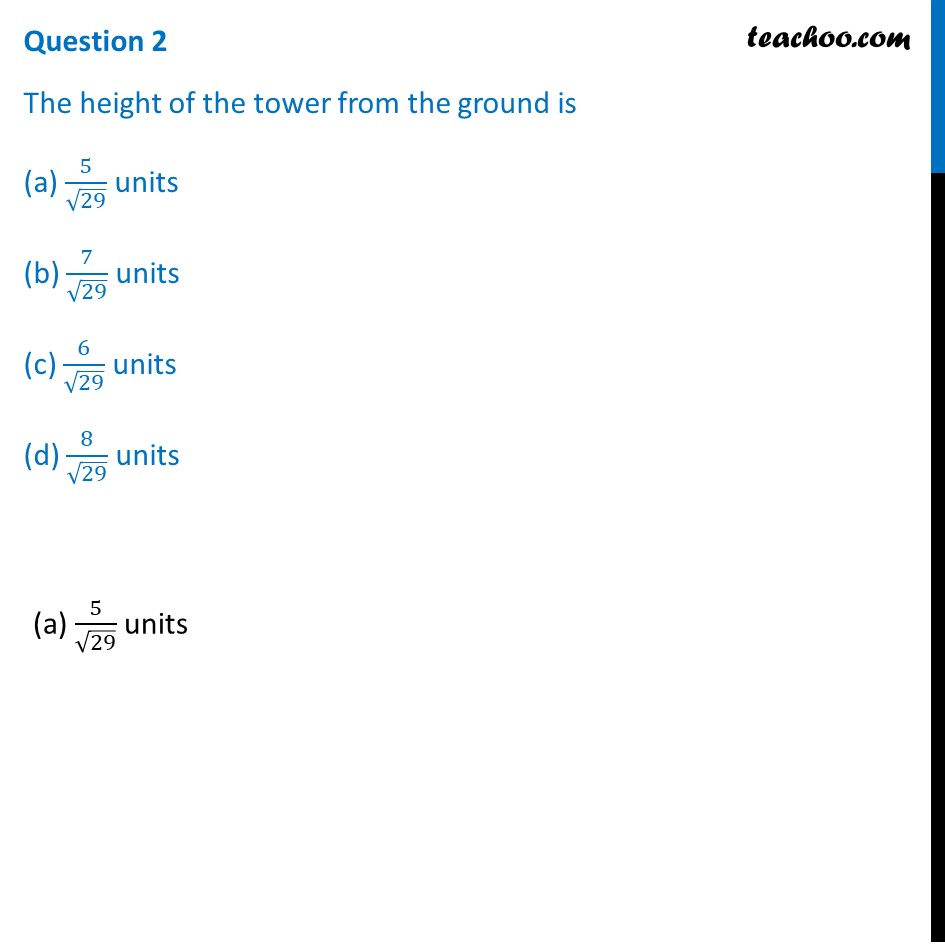## (d) (x + 1)/(-2)=(y + 3)/(-1)=(z - 5)/2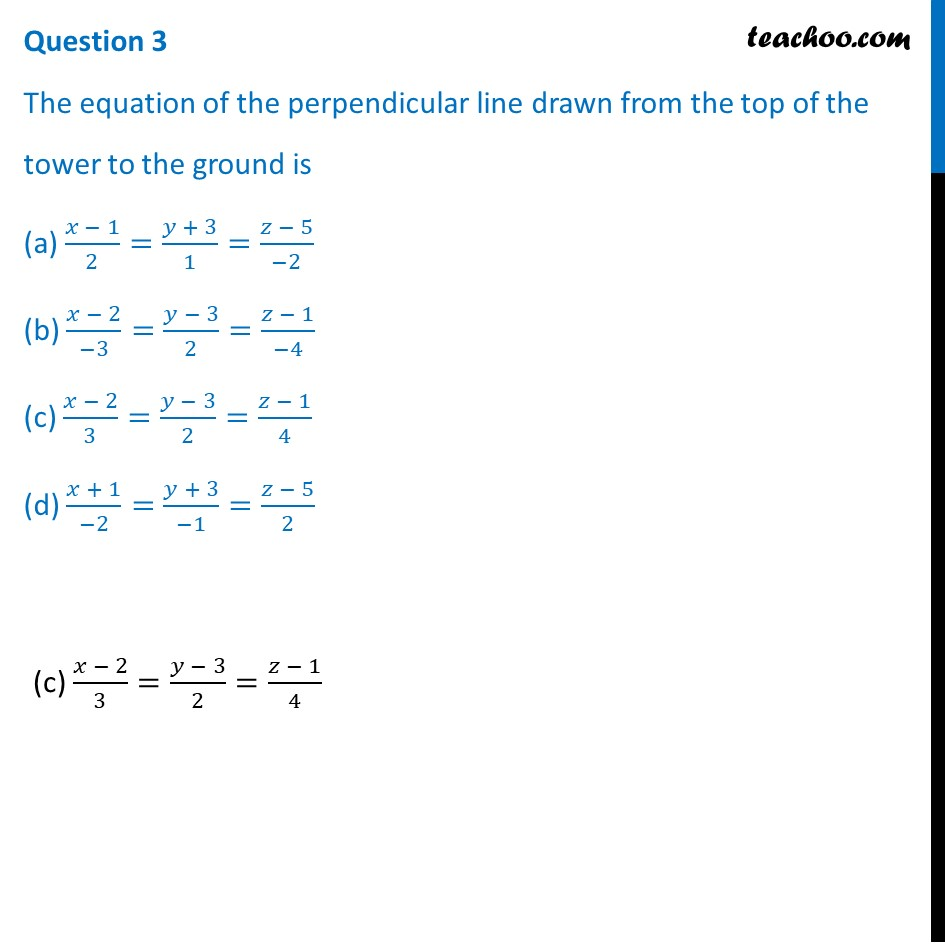## (d) (43/29,77/29,9/29)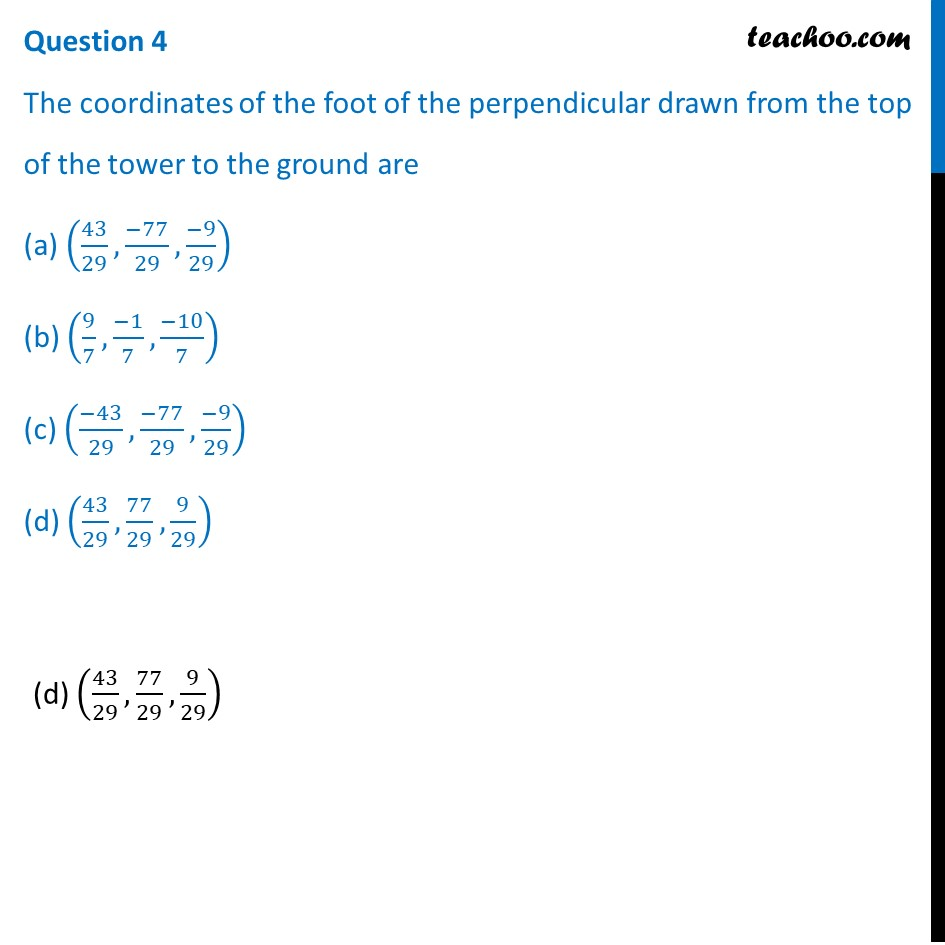## (d) √39/4 units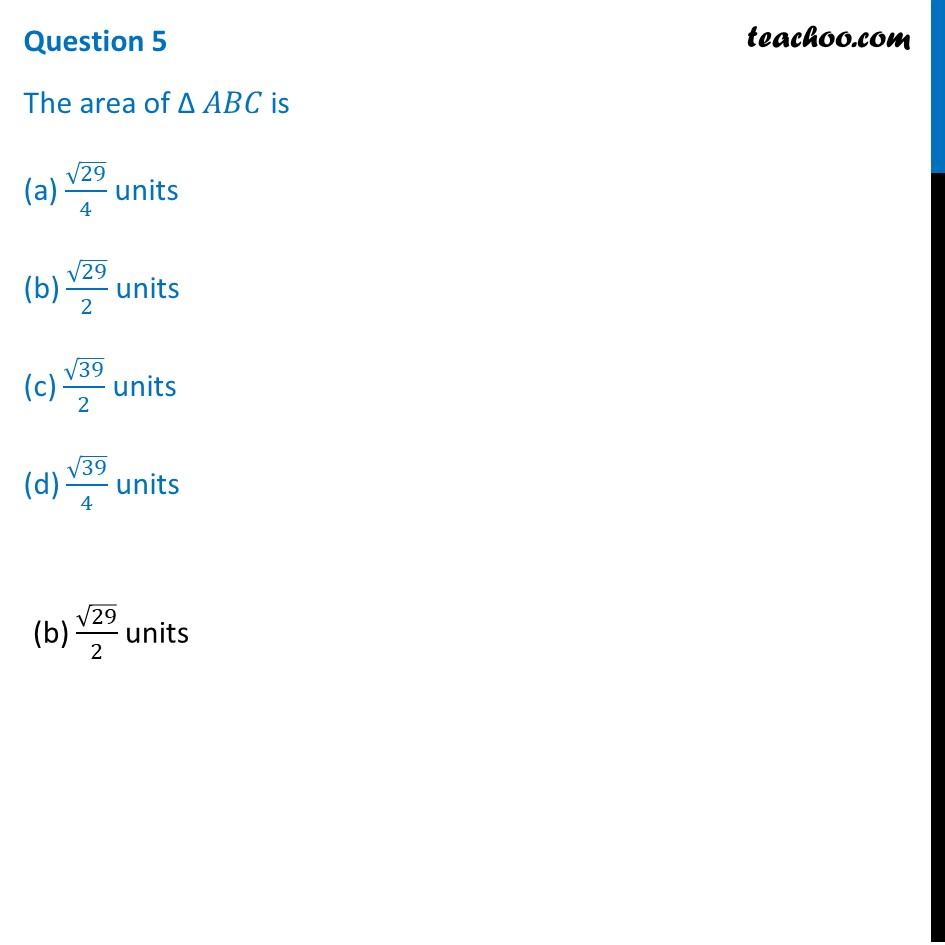1. Chapter 11 Class 12 Three Dimensional Geometry (Term 2)
2. Serial order wise
3. Case Based Questions (MCQ)

Transcript

Question A mobile tower stands at the top of a hill. Consider the surface on which the tower stands as a plane having points A (1, 0, 2), B (3, –1, 1) and C (1, 2, 1) on it. The mobile tower is tied with 3 cables from the point A, B and C such that it stands vertically on the ground. The top of the tower is at the point (2, 3, 1) as shown in the figure. Based on the above answer the following:Question 1 The equation of the plane passing through the points A, B and C is (a) 3x – 2y + 4z = –11 (b) 3x + 2y + 4z = 11 (c) 3x – 2y – 4z = 11 (d) –3x + 2y + 4z = –11(b) 3x + 2y + 4z = 11 Question 2 The height of the tower from the ground is (a) 5/√29 units (b) 7/√29 units (c) 6/√29 units (d) 8/√29 units(a) 5/√29 units Question 3 The equation of the perpendicular line drawn from the top of the tower to the ground is (a) (𝑥 − 1)/2=(𝑦 + 3)/1=(𝑧 − 5)/(−2) (b) (𝑥 − 2)/(−3)=(𝑦 − 3)/2=(𝑧 − 1)/(−4) (c) (𝑥 − 2)/3=(𝑦 − 3)/2=(𝑧 − 1)/4 (d) (𝑥 + 1)/(−2)=(𝑦 + 3)/(−1)=(𝑧 − 5)/2 (c) (𝑥 − 2)/3=(𝑦 − 3)/2=(𝑧 − 1)/4 Question 4 The coordinates of the foot of the perpendicular drawn from the top of the tower to the ground are (a) (43/29,(−77)/29,(−9)/29) (b) (9/7,(−1)/7,(−10)/7) (c) ((−43)/29,(−77)/29,(−9)/29) (d) (43/29,77/29,9/29)(d) (43/29,77/29,9/29) Question 5 The area of ∆ 𝐴𝐵𝐶 is (a) √29/4 units (b) √29/2 units (c) √39/2 units (d) √39/4 units(b) √29/2 units

Case Based Questions (MCQ)